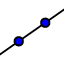# Basic Geometric Postulates Part 2

The figure below shows two planes - one purple and one pink. Click on the move arrow(you have to choose it twice) and then click on empty white space in the diagram below and click and hold your mouse and drag the arrow around to change your view of the two planes. As you do so, focus on which points are (a) on just the pink plane; (b) on just the purple plane; (c) on both planes; and (d) on neither plane. Also focus on the intersection of the two planes.

If I asked you to identify plane KFE, which plane would that be? Explain in a few sentences why naming a plane with those three letters might be a problem (HINT: if you are stuck go back and look at your notes for the naming rules for planes that we learned).

## Postulate #4: Plane Intersection Postulate

Focus on the intersection of the purple and pink planes. What statement below accurately describes what you see? ONLY CHOOSE ONE ANSWER.

Select all that apply
• A
• B
• C

In the diagram above, which points do not lie on either plane?

Select all that apply
• A
• B
• C
• D
• E
• F
• G
• H

In the diagram above, which points lie on both planes?

Select all that apply
• A
• B
• C
• D
• E
• F
• G
• H

In the diagram above, which points lie on the pink plane?

Select all that apply
• A
• B
• C
• D
• E
• F
• G
• H
Now lets add a few line elements to the existing drawing.

These questions refer to the above applet. Points are "collinear" if a line COULD be drawn through the named points (the line does not have to actually be there). With that in mind, which of the following are collinear? If you aren't sure, use the line toolto see if a line can be drawn between the two points (click the line tool first THEN click on the two points that you want to draw a line through).

Select all that apply
• A
• B
• C
• D
• E
• F
• G
Now lets try drawing a plane. Click on the plane drawing tooland then click on three points H, D and I.

## Postulate #5: Three Points Postulate

When you clicked on three points, a plane appeared in the applet. Is there any other plane that goes through those three points? What statement below accurately describes the situation you see above.

Select all that apply
• A
• B
• C

Plane HDI (the new plane you created) intersects plane AFB where?

Select all that apply
• A
• B
• C
• D